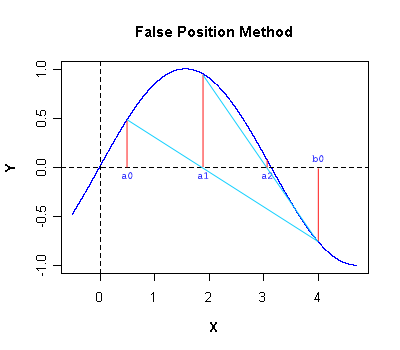I have forgotten

•http://facebook.com/
•https://www.google.com/accounts/o8/id
•https://me.yahoo.com
COST (GBP)2.980.000

# Falseposition

Calculates the zeros of a function using the Regula-Falsi method.
Controller: CodeCogsC++

## Falseposition

 doublefalseposition( double (*f)(double)[function pointer] double x0 = -1E+7 double x1 = 1E+7 double eps = 1E-10 double maxit = 1000 )
The false position method, on contrast with Newton's method, in which the calculation of the derivative was required, replaces it with its approximation

Given and , the main algorithm is based on the following recurrence relation

This method has rate of convergence , therefore inferior to the method of Newton. However it is more accurate than Newton because only one function evaluation is required, , being calculated in the previous iteration, while Newton requires two, and .

To give you a better idea on the way this method works, the following graph shows different iterations in the approximation process. Here is the associated list of pairs chosen at consecutive stepsThis algorithm finds the roots of the user-defined function f starting with an initial interval [x0, x1] and iterating the sequence above until either the accuracy eps is achieved or the maximum number of iterations maxit is exceeded.

## Example:

#include <codecogs/maths/rootfinding/falseposition.h>

#include <iostream>
#include <iomanip>
#include <cmath>

// user-defined function
double f(double x) {
return cos(x);
}

int main()
{
double x = Maths::RootFinding::falseposition(f, 1, 3);

std::cout << "The calculated zero is X = " << std::setprecision(12) << x << std::endl;
std::cout << "The associated ordinate value is Y = " << f(x) << std::endl;
return 0;
}
Output:
The calculated zero is X = 1.70614146372
The associated ordinate value is Y = -0.134932299622

## References:

• F.R. Ruckdeschel, "BASIC Scientific Subroutines", Vol. II, BYTE/McGRAWW-HILL, 1981

### Parameters

 f the user-defined function x0 Default value = -1E+7 x1 Default value = 1E+7 eps Default value = 1E-10 maxit Default value = 1000

### Authors

Lucian Bentea (August 2005)
##### Source Code

Source code is available when you buy a Commercial licence.

Not a member, then Register with CodeCogs. Already a Member, then Login.